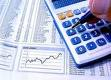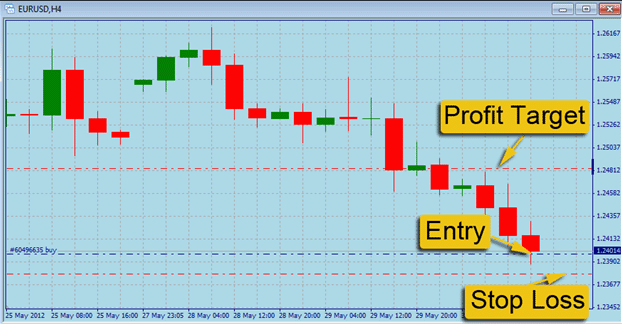»» Calculating Profits and Losses in the forex.

## Calculating Profits and Losses in the forex.

Calculating Profit / Loss (Gain / Loss)The movement of units / lowest price in the forex is calculated in units of points / pips. The value of each point will vary according to type of currency pairs (pair).

## Calculating Profit Loss Based Account Types

Total contract size used is in units of lots, namely:– Standard lot (\$ 100,000)

– Mini lot (\$ 10,000)

– Micro lot (\$ 1,000)

### Pair To Direct Rates

Direct rates USD pair is the pair with a suffix (GBP / USD, EUR / USD, AUD / USD, and NZD / USD), the way the calculations.

Profit / loss = (Selling Price – Purchase Price) x contract size x lot.Example:

Buy 4 standard lot EUR / USD 1.2500 and Sell 4 standard lot EUR / USD 1.2570

Profit = (1.2570 – 1.2500) x 100,000 x 4

Profit = \$ 2,800

Sell ​​1 standard lot of GBP / USD 2.0010, Buy 1 standard lot of GBP / USD 2.0000

Profit = (1.2010 – 1.2000) x 100,000 x 1

Profit = \$ 100

There are easy ways to pair the calculation of which end in USD is:

gain 1 point for 1 standard lot (100,000) is \$ 10.

gain 1 point for 1 mini lot (10,000) is \$ 1

gain 1 point for 1 micro lot (1,000) is \$ 0.1

### For Indirect Rates Pair

Indirect rates pairs are pairs with USD as the prefix (USD / JPY, USD / CHF, and USD / CAD), how to calculate profit / loss are as follows:

Profit / loss = (Selling Price – Purchase Price) / Price Liquidation x contract size x lot.Example:

Buy 1 standard lot USD / JPY 110.00

Sell ​​1 standard lot USD / JPY 110.05

Profit = (110.05 – 110.00) / 110.05 x 100,000 x 1 = \$ 45.43

### For Cross Currency Rates

Pair that do not contain the USD (GBP / JPY, EUR / JPY, AUD / JPY, EUR / GBP, and GBP / CHF) calculation of profit / loss are as follows:

Profit = (Selling Price – Purchase Price) x Rate Base Currency Current / Rate Pair x contract size x lot

Example:

Sell ​​1 lot of the standard EUR / GBP at 0.6760 price

(EUR / USD is the base currency of EUR / GBP, because the front of the EUR / GBP is the Base Currency)

Buy 1 standard lot EUR / GBP at 0.6750 price Rate EUR / USD: 1.1840

Profit = (0.6760 – 0.6750) x 1.1840 / 0.6750 x 100,000

Profit = \$ 175.4

#### Margin and Leverage

The term margin leverage in the forex margin trading means that if you want to trade for \$ 10,000, you do not have to provide \$ 10,000 but \$ 100 is enough to provide meaningful leverage 1:100. As a guarantee fund to your broker. Leverage the value of this variety is usually in the ratio 1:50, 1:100, and 1:200

So like you have the cash \$ 1,000 in broker who has the leverage to 1:100. This means you can trade with the amount of up to approximately \$ 100,000 (or nearly 100 x folding your capital). This also means that to use the contract size of \$ 100,000.

The advantage of the leverage is with a smaller capital you can trade with a total contract size / lot the same as if you do not use leverage. Or it can be said, with equal capital, you can use the contract size is greater than not using the leverage. So with the same capital, you have the opportunity to increase profit per pip more better.

Professional Forex Brokers That Have Been Tested And Recommended To Trading Forex :